23年专注环保水处理设备研发制造 环保设备系统设计\制作\安装一条龙服务

# 【干货】 | 格栅、污泥池、风机、MBR、AAO进出水系统的计算公式1、栅隙
(1)水泵前格栅栅条间隙应根据水泵要求确定。
(2) 废水处理系统前格栅栅条间隙，应符合下列要求：最大间隙40mm，其中人工清除25~40mm，机械清除16~25mm。废水处理厂亦可设置粗、细两道格栅，粗格栅栅条间隙50~100mm。
(3) 大型废水处理厂可设置粗、中、细三道格栅。
(4) 如泵前格栅间隙不大于25mm，废水处理系统前可不再设置格栅。
2、栅渣
(1) 栅渣量与多种因素有关，在无当地运行资料时，可以采用以下资料。

(2) 栅渣的含水率一般为80%，容重约为960kg/m3
(3) 在大型废水处理厂或泵站前的大型格栅（每日栅渣量大于0.2m3)，一般应采用机械清渣。
3、其他参数
(1) 过栅流速一般采用0.6~1.0m/s。
(2) 格栅前渠道内水流速度一般采用0.4~0.9m/s。
(3) 格栅倾角一般采用45°~75°，小角度较省力，但占地面积大。
(4) 机械格栅的动力装置一般宜设在室内，或采取其他保护设备的措施。
(5) 设置格栅装置的构筑物，必须考虑设有良好的通风设施。
(6) 大中型格栅间内应安装吊运设备，以进行设备的检修和栅渣的日常清除。

1、平面格栅设计计算
(1) 栅槽宽度B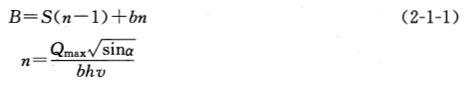(2) 过栅水头损失如(3) 榭后槽总高H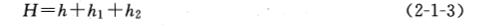(4) 栅槽总长L(5)每日栅渣量W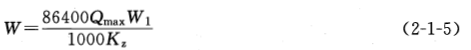1、基底压力计算
(1)水池自重Gc计算

(2)池内水重Gw计算

(3)覆土重量计算

(4)活荷载作用Gh

(5)基底压力Pk

=(945.00+721.50+279.50+45.50+119.00)/42.500= 49.66 kN/m2
2、修正地基承载力
(1)计算基础底面以上土的加权平均重度rm
rm=[1.000×(20.00-10)+2.000×18.00]/3.000
= 15.33 kN/m3
(2)计算基础底面以下土的重度r

(3)根据基础规范的要求，修正地基承载力:
fa = fak + ηb γ(b - 3) + ηdγm(d - 0.5)
= 100.00+0.00×10.00×(5.000-3)+1.00×15.33×(3.000-0.5)
= 138.33 kPa
3、结论
Pk=49.66<fa=138.33 kPa, 地基承载力满足要求。

Gk/F=1270.00/425.00=2.99 > Kf=1.05, 抗浮满足要求。

1、顶板荷载计算:

Qt = 1.20×P1 + 1.27×Ph= 7.91 kN/m2

Qte = P1 + 0.40×Ph= 5.60 kN/m2
2、池壁荷载计算: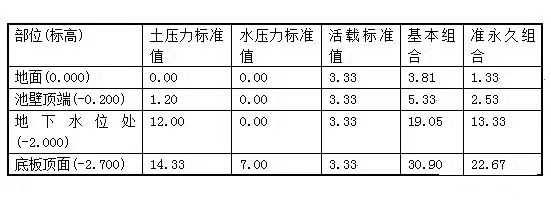3、底板荷载计算(池内无水，池外填土)：

Qb = (945.00×1.20+279.50×1.27+45.50×1.27+119.00×1.27×0.90)/42.500
= 39.59kN/m2

Qbe = (945.00+279.50+45.50×1.00+1.50×36.000×0.40+10.00×6.500×0.40)/42.500
= 31.00kN/m2

Q = 39.59-0.300×25.00×1.20= 30.59 kN/m2

Qe = 31.00-0.300×25.00
= 23.50 kN/m2
4、底板荷载计算(池内有水，池外无土):

Qb=[4.500×8.000×1.50×1.27+945.00×1.20+(3.900×7.400×2.500)×10.00×1.27]/42.500
= 49.86kN/m2

Q = 49.86-(0.300×25.00×1.20+2.500×10.00×1.27) = 9.11kN/m2

Qbe=[4.500×8.000×1.50×0.40+945.00+(3.900×7.400×2.500)×10.00]/42.500
= 39.72kN/m2

Qe=39.72-(0.300×25.00+2.500×10.00)
= 7.22kN/m2

1、弯矩正负号规则

2、荷载组合方式
1.池外土压力作用(池内无水，池外填土)
2.池内水压力作用(池内有水，池外无土)
3.池壁温湿度作用(池内外温差=池内温度-池外温度)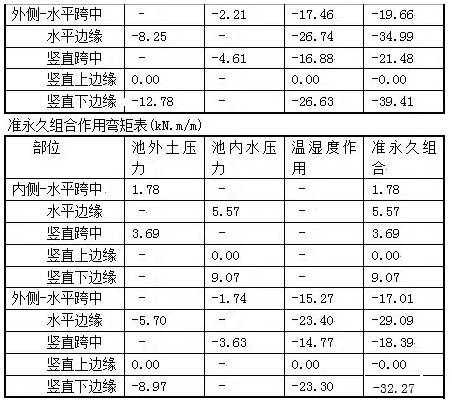B侧池壁内力：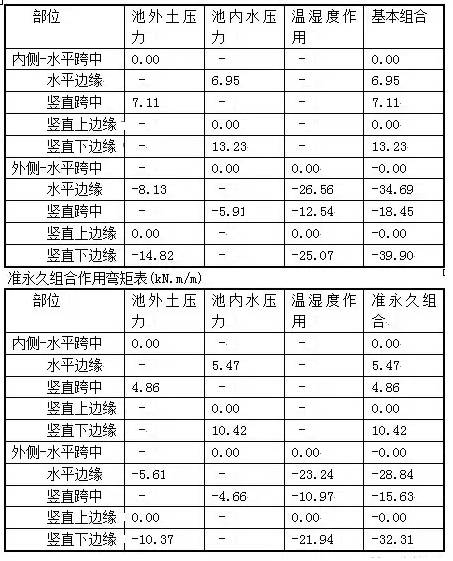1、池外填土，池内无水时，荷载组合作用弯矩表(kN·m/m)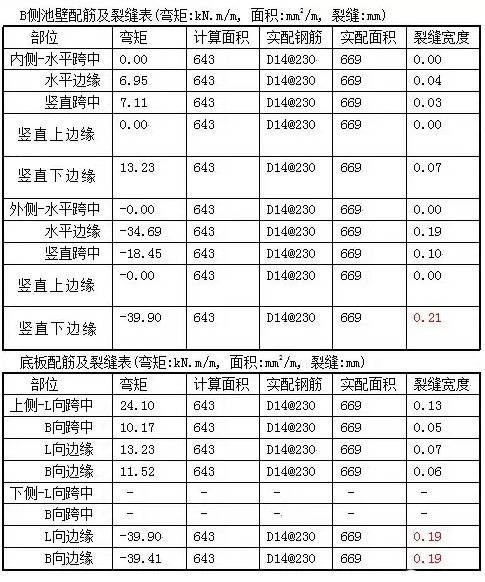(简化，近似，一般情况下用)

1、轴功率：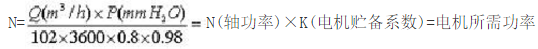2、风机全压：(未在标准情况下修正)(760mmHg)－(海拨高度÷12.75)=当地大气压 (mmHg)

1mmH2O=9.8073Pa
1mmHg=13.5951mmH2O
760mmHg=10332.3117 mmH2O

1000～1500M海拨高度时加2%的流量；
1500～2500M海拨高度时加3%的流量；
2500M以上海拨高度时加5%的流量。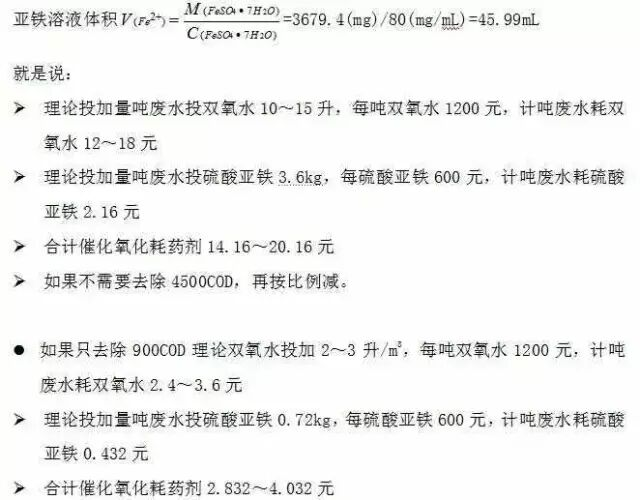MBR计算公式## AAO进出水系统设计计算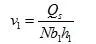b1——进水渠道宽度(m)；
h1——进水渠道有效水深(m)。

V1=0.66/(2×1.0×1.0)=0.33m/s

F=Qs/Nv2

v2——孔口流速(m/ s )，一般采用0.2～1.5 m/ s 。

F=0.66/2×0.4=0.66m2

N=F/f

f——每个孔口的面积( m2 )。
n=0.66/0.5×0.5=2.64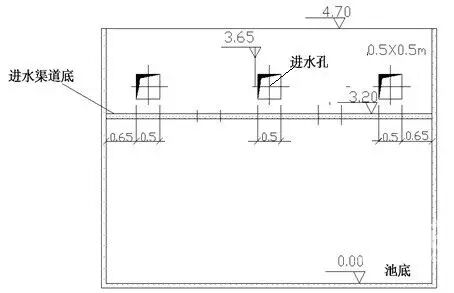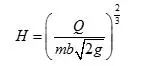Q——每座反应池出水量(m3/s)，指污水最大流量( 0.579m/s)；与回流污泥量、回流量之和(0.717×160% m3/s)；
m——流量系数，一般采用0.4～0.5；
b——堰宽(m)；与反应池宽度相等。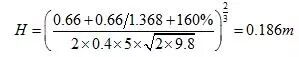------------------------------------------